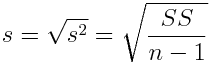The standard deviation is the most frequently reported measure of variability for numerical variables. Think of it as a typical value of deviations from the mean for the data set. It is calculated by taking the square root of the variance.Thus, the standard deviation is in the same units of measurement as the data. If the data are measurements in inches, then the standard deviation is also in inches (as is the mean and median). Return To Main Page